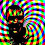## Sunday, October 26, 2008

### The Shuffle

The shuffle, also known as the Fisher's Exact Test, is a permutation test that can be used to estimate the sampling distribution of a statistic without relying on parametric assumptions. This is especially important when sample sizes are small. The other neat thing about permutation tests is that you don't have to know what the distribution of your statistic is. So if you have a really odd function of your data that you want to use rather than one of the classical statistics you can.

Octave has a great built-in function called nchoosek which makes shuffling a breeze. Called with scalar arguments it returns the value of the binomial coefficient, which is the number of ways you can choose k things from n things (k<=n). For fixed n, nchoosek is maximum when k=n/2. That is plotted below for n=2:24.Notice that's a log-log scale, as n increases it quickly becomes intractable to perform a complete permutation test.

Suppose we have two data vectors, and we want to know if they are from populations with different means. We can use the normrnd() function to draw 8 samples from a normal distribution with 0 mean and 8 samples from a normal distribution with a mean of 1.

n=8;
x1 = normrnd(0,1,n,1);
x2 = normrnd(1,1,n,1);
perms = nchoosek( [x1;x2], n );

We stored all 12870 permutations of the vectors (16 choose 8) in the matrix perms. Now we use the built-in function complement to find the difference of the means under each of those labellings.

for(i=1:length(m1) )
comps(i,:) = complement( perms(i,:), [x1;x2] );
endfor
m1 = mean( perms, 2 ); % take row-wise averages, DIM=2
m2 = mean( comps, 2 ); % take row-wise averages, DIM=2
m_shuff = m1 - m2;

Now we can use the distribution of m_shuff to decide if the difference in the means of our two data vectors is significant.Octave script with all of these calculations: shuffle.m.

#### 1 comment:

1.What about the bootstrap you say? That's really easy in Octave too:

x = empirical_rnd( n, data );

Draws a bootstrap sample (that's with replacement) of size n from your data vector.